# Calcularing area vector using line integral

• SquidgyGuff

## Homework Statement

A closed curve C is described by the following equations in a Cartesian coordinate system: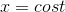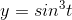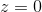where the parameter t runs monotonically from 0 to 2π, thus defining the direction of C. Calculate the area vector of the planar region enclosed by C, using the formula: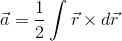2. The attempt at a solution
I'm mostly having trouble defining what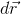is as a physical quantity. I think it is the distance to an incremented point along the curve such that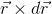it the area of the equilateral shape formed by the vectors and that half the integral of that gives the area but I'm suck here: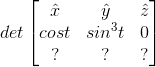Than I can evaluate it as: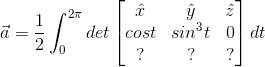and this would give a result that is only in the z direction which dimensionally makes sense

## Homework Statement

A closed curve C is described by the following equations in a Cartesian coordinate system:where the parameter t runs monotonically from 0 to 2π, thus defining the direction of C. Calculate the area vector of the planar region enclosed by C, using the formula:2. The attempt at a solution
I'm mostly having trouble defining whatis as a physical quantity. I think it is the distance to an incremented point along the curve such thatit the area of the equilateral shape formed by the vectors and that half the integral of that gives the area but I'm suck here:Than I can evaluate it as:and this would give a result that is only in the z direction which dimensionally makes sense

If
$$\vec{r} = x(t) \, {\bf i} + y(t) \, {\bf j} + z(t)\, {\bf k}$$
how would you compute ##d \vec{r}## in terms of ##dt##?

If
$$\vec{r} = x(t) \, {\bf i} + y(t) \, {\bf j} + z(t)\, {\bf k}$$
how would you compute ##d \vec{r}## in terms of ##dt##?
My first instinct was just to derive each of them with respect to t like such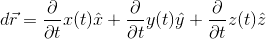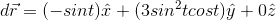Is that right?

My first instinct was just to derive each of them with respect to t like suchIs that right?
Yes, go ahead and finish the evaluation of the integral ...

Yes, go ahead and finish the evaluation of the integral ...
So http://www.sciweavers.org/upload/Tex2Img_1442441349/eqn.png [Broken] (by trig identities)
and so the integral is

Last edited by a moderator:
So http://www.sciweavers.org/upload/Tex2Img_1442441349/eqn.png [Broken] (by trig identities)
and so the integral is